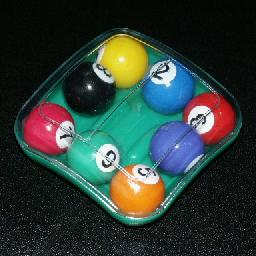# Diamond 8-Ball PuzzleThis puzzle consists of 8 numbered balls which can roll around a 3x3 square. There is a restriction that differentiates it from a simple 3x3 version of the fifteen puzzle. There are walls on the left and right side of the centre position, so that a ball can enter or leave the centre in a vertical direction only. In the solved state the centre spot is empty, and balls are in clockwise numerical order starting from 1 at the top left corner.

This puzzle was invented by Joshua Frankel, and manufactured by Binary Arts, now called ThinkFun.

If your browser supports JavaScript, then you can play Diamond Eightball by clicking the link below:

## The number of positions:

If we count only those positions with the gap in the centre, then there are 8 pieces which can be any order, giving a maximum of 8! = 40,320 positions. As with the fifteen puzzle, only even permutations are achievable, so there are actually 8!/2 = 20,160 positions with the centre empty, or 9!/2 = 181,440 positions with the empty spot anywhere.
Note however that there are really two solutions - you could solve it with the 1 ball at the bottom right, and then turn the puzzle upside down.

I performed a computer search for this puzzle. The following table shows how many positions there are (with the centre empty) for each number of moves from the solved position. A move consists of moving the space around the left or right side in either direction, shifting five pieces in the process. The first column shows the number of positions at each distance from only one of the two solutions, the second if either solution is allowed.

Moves    One solution    Two solutions
012
148
21224
33264
482156
5204360
6496792
71,1531640
82,4313202
94,3255132
105,8295556
114,4082916
121,133306
13502
Total   20,160   20,160
Avg Depth9.51969.0299

## Notation:

There are 2 loops in the puzzle; the left half and the right half. Denote these by L and R. A clockwise shift of all the five balls in a loop is indicated by the relevant letter (L or R), and an anti-clockwise shift by the letter followed by an apostrophe (i.e. by L' or R').

## Solution:

Phase 1: Solve balls 3,4,5, and 6.

1. Do any moves to bring ball 5 to the top right corner, i.e. the position where 3 will be when solved.
2. If ball 4 lies on the right (directly below the 5, or in the bottom right corner), then do RR LL R'R' so that 4 lies in the left loop and 5 is again top right.
3. Shift the left loop to bring ball 4 to the top position, and then do R. You now should have ball 4 at the top right, and 5 directly below it.
4. If ball 3 lies at the bottom right corner, then do R L R'.
5. Shift the left loop to bring ball 3 to the top position, and then do R. You now should have balls 3, 4 and 5 in their correct positions.
6. Shift the left loop to bring ball 6 to the bottom position, where it belongs.

Phase 2: Solve the rest.

1. Find ball 7. Depending on its position, do one of the following sequences:
top-left: L'RL'R' LLRL'L'R'
top: RLL R'LR LLR'
left: R'LRLL R'L'L'RL
2. Find ball 8. Depending on its position, do one of the following sequences:
top-left: LR'L'RL'R'LLRL
top: L'R'L'RL'R'LLRL'

## Nice sequences:

LRLRLRL: Constructs the second solution, with the 1 at the bottom right corner.